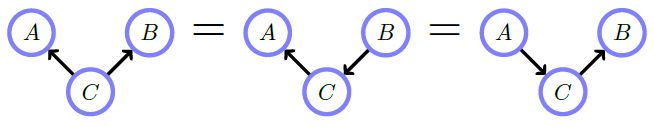Math and science::INF ML AI

# Belief networks: independence examples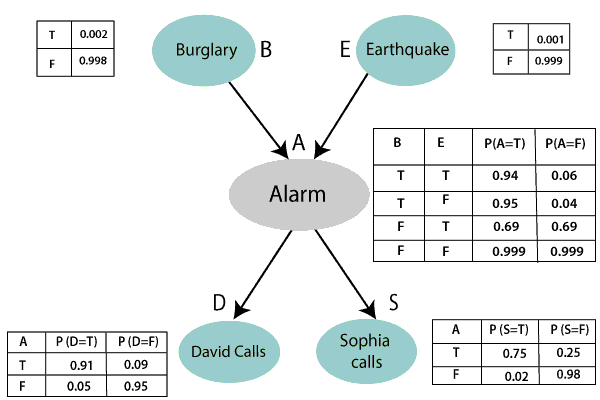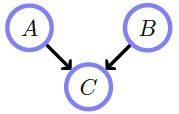1 a) Marginalizing over $$C$$  makes $$A$$ and $$B$$ [...]

1 b) Conditioning on $$C$$ makes A and B [...]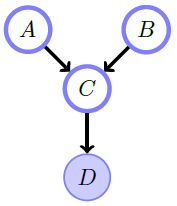2. Conditioning on $$D$$, a descendent of a collider $$C$$ makes $$A$$ and $$B$$  [...]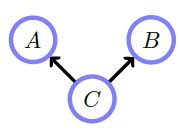3 a) $$p(A, B, C) =$$ [...]

3 b) Marginalizing over $$C$$ makes $$A$$ and $$B$$ [...]

3 c) Conditioning on $$C$$ makes $$A$$ and $$B$$  [...]

Finally, these following graphs all express the same conditional independence assumptions.